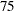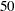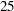Summary Statistics Represented by Box Plots

Table 25.6 lists the summary statistics represented in each box-and-whiskers plot.

Table 25.6 Summary Statistics Represented by Box Plots

Group Summary Statistic

Feature of Box-and-Whiskers Plot

maximum

endpoint of upper whisker

third quartile (th percentile)

upper edge of box

median (th percentile)

line inside box

mean

symbol marker

first quartile (th percentile)

lower edge of box

minimum

endpoint of lower whisker

Note that you can request different box plot styles, as discussed in the section Styles of Box Plots, and as illustrated in Example 25.2.